# Exercise 2: Add User Subroutines

In this step, you will add Python user subroutines to the MotionView model.

Python can use many MotionSolve functions and inputs when certain syntax rules are followed. When using a MotionSolve function such as AKISPL or SYSFNC, the string “py_” must be added to the beginning. For example, “py_sysfnc (…” would be the correct usage of SYSFNC in Python. When defining a usersub function in Python, the name of the function and the inputs must match those outlined in the MotionSolve online help pages exactly. When accessing model data in Python through a function such as SYSFNC, use the exact property name in quotations as the “id” input. Model properties that are passed into Python in the function definition can be accessed throughout the script, and do not need additional defining to use. An example of these syntax rules being used is shown below:
def REQSUB(id, time, par, npar, iflag):
[A, errflg] = py_sysfnc(“DX”,[par,par])
return A

## Use SPLINE_READ to Replace the Curve Entity

In this step you will implement the SPLINE_READ subroutine.

This user-subroutine uses the SPLINE_READ function to return the curve from the included pressure_curve.csv file. SPLINE_READ is the usersub that corresponds to the curve entity in MotionView. It uses data points in an external file to create a curve, which can then be used by other entities.

Write the Python script:

1. Open a new Python file.
2. Define a function with the name SPLINE_READ using “def SPLINE_READ():", and give the inputs and outputs id, file_name, and block_name.
3. Import the Python CSV package by including import csv after the function definition.
4. Open pressure_curve.csv in the function, and read the file to your Python script as a variable. Use the function “variable = open(‘pressure_curve.csv’,’r’)”.
5. Define a new variable to change the format of this variable. Use csv.reader() to read your variable file.
6. Define an empty list, “L”, to store the pressure_curve data values. Iterate through the list using “for item in curv:”. Append each item as a separate list value with “L.append(item)”.
7. Remove the headers from the csv file by redefining the list from the second value till the end of the list. This can be done with “L = L[1:]”.
8. Define a counter variable to be used later.
9. Define two lists that are half the length of “L”, and set them equal to zero.
1. Use “x = 16*[0.0]” twice; once with the x value and once with the y value.
10. Create a while loop dependent on your counter variable being less than the length of your list, minus one.
11. In each iteration of the loop, define your x and y data values for the index “i” as a floating value of each half of your “L” data sets. This should look like “x[i] = float(L[i])” and “y[i] = float(L[i])”. Increase your counter variable by 1.
12. Define a z variable with a floating value of 0.0, and close the csv file.
Note: Defining a z variable is necessary, as the next function you will use requires an x, y, and z variable.
13. Use the put_spline MotionSolve function, and return the “id”, as well as the lists containing the first and second column of values and the z variable. This should be done with “errflg = py_put_spline(id,x,y,z)” followed by “return errflg”.
14. Save the file to your <working directory> as nonlin_spline.py.
Your script should resemble the following example:
def SPLINE_READ(id, file_name, block_name):
import csv
ifile= open('pressure_curve.csv','r') ## opens data file as
useable var.
L = [] ## creates empty list
for item in curv:
L.append(item) ## separates file values into list
L = L[1:] ## removes block names from list
i=0 ## creates counter
x = 16*[0.0]
y = 16*[0.0] ## splits list into x and y lists
while i < (len(L)-1):
x[i] = float(L[i]) ## changes values from str to float
y[i] = float(L[i])
i+=1 ## counter increment
z = 0.0 ## defines z value
ifile.close() ## closes data file
errflg = py_put_spline(id,x,y,z) ## var to create MotionSolve
spline
return errflg ## returns var
15. Implement the Python script:
1. In MotionView, click the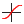(Curve) button.
2. Locate the Force_Pressure curve in the Project Browser.
3. From the Properties tab, check the box marked User-defined.
4. From the Attributes tab, make sure Linear extrapolation is checked.
5. Click on the User-Defined tab, and use the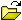(File browser) to select the pressure_curve.csv file.
6. Change the Function Type in the drop-down menu from DLL to Python, and ensure the function name is SPLINE_READ. You do not need to enter anything for the Block name, as it is not needed in this tutorial.
7. Check the box marked Use local file and function name. Use the(File browser) to locate and select the nonlin_spline.py file.

## Use REQSUB to Request an Output

In this step, you will use the REQSUB user-subroutine to return values of the magnitude of displacement for the piston.

This subroutine will use Python to specify which values to return.

Write the Python script:

1. Create a new Python file and define a function named REQSUB with the appropriate inputs and outputs.
This is the syntax: “def REQSUB(id, time, par, npar, iflag)”.
2. Use the sysfnc utility to implement the “DM” (displacement magnitude) function on the first and second input parameters, and define a variable and an error flag by writing “[D, errflg] = py_sysfnc(“DM”,[par,par])”.
3. Return a list of eight values, where the second value is your variable, and the rest are equal to 0.
This will be your result variable, and should look like “result = [0,D,0,0,0,0,0,0]”.
4. Save this file to your <working directory> as req_nonlin.py.
Your script should resemble the following example:
def REQSUB(id, time, par, npar, iflag):
[D, errflg] = py_sysfnc("DM",[par,par]) ## sets "D" as piston
displacement mag
result = [0,D,0,0,0,0,0,0] ## lists results for output
return
return result ## sends list with results to motionsolve as
output
5. Implement the Python script.
1. In MotionView, click on the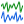(Outputs) panel.
2. Locate the Output_Conrod_Length in the Project Browser.
3. From the Outputs panel, from the drop-down menu select User defined.
4. Click in the text field labeled Output, and then click on the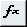button to open the Expression Builder. In the text field, add two sets of curly brackets (“{},{}”).
5. From the Joints folder, click idstring. Click inside the first set of brackets and click Add to insert j_0.i.idstring.
6. Click inside the second set of brackets and add the next expression, j_1.i.idstring.
7. Click OK.
8. Check the Use local file and function name box, and select Python from the Function Type drop-down menu.
9. Use the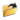(Local File) file browser to locate and select the req_nonlin.py script, and make sure the Function name text field reads REQSUB.

## Use GFOSUB to Replace the Force Entity

In this step, you will replace the Force Entity with a user defined Python script using GFOSUB.

The GFOSUB used here will take the curve data defined with SPLINE_READ, and change depending on the Conrod angle according to the curve.

Write the Python script:

1. Open a new Python file.
2. Define the function GFOSUB by typing “def GFOSUB(id, time, par, npar, dflag, iflag):”.
3. Import "pi" from the Python “math” library using “from math import pi”.
4. Use the “AZ” function for angle in the z direction with the sysfnc command, to save it as a variable. To do this, type “[A, errflg] = py_sysfnc(“AZ”,[par,par])”.
5. Change the variable defined in Step 4 to degrees. As the model extends from the origin into the negative y direction, you will need to multiply by -1.
Note: The method used in this tutorial is “B = ((-1)*A*180)/pi”
.
6. Define another variable using the “akispl” utility, which interpolates the force values from the curve. You will need input arguments of your angle “B”, zero to specify a two dimensional curve, and zero for the curve input and the order.
Note: This line is written as “[C, errflg] = py_akispl(B,0,par,0)”.
7. Return a list three elements long, where the second element is the variable defined with the Akima interpolation function. The data from interpolation is stored in the first column, so use “return [0,C,0]”.
8. Save this file to your <working directory> as gfo_nonlin.py.
Your script should resemble the following example:
def GFOSUB(id, time, par, npar, dflag, iflag):
from math import pi
[A, errflg] = py_sysfnc("AZ",[par,par]) ## retreives conrod
angle
B = ((-1)*A*180)/pi ## converts radians
to degrees
[C, errflg] = py_akispl(B,0,par,0) ## interpolates data to fit
curve
return [0,C,0] ## returns C data as force values
9. Implement the Python script.
1. In MotionView, click the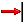(Force) icon.
2. Locate the Force_Gas_Pressure force in the Project Browser.
3. From the Connectivity tab, check the User-defined properties box.
4. From the User-Defined tab, edit the Force value with the Expression Builder to include the curve idstring, the ground marker idstring, and the crank marker idstring.
5. Click OK.
6. Check the Use local file and function name box, and select Python from the Function Type drop-down menu.
7. Useto select gfo_nonlin.py from your <working directory>.
Remember: Make sure the Function name is set to GFOSUB.

## Use MOTSUB to Define a Motion

In this step you will use the MOTSUB user subroutine to define a motion.

Write the Python script:

1. Open a new Python file.
2. Define the MOTSUB function, including the required inputs. The correct syntax for this is “def MOTSUB(id, time, par, npar, iord, iflag):”.
3. The MOTSUB user subroutine requires a function or expression, and its first and second order derivatives. Create conditional statements using the function order variable “iord” to define the function and its first and second derivatives with “if iord==0:”, “elif iord==1:” and “else:”.
4. The function and its derivatives should be defined with the same variable name. The function used in this tutorial is “A = 10.0461*time”. This makes the first derivative equal to “A = 10.0461”, and the second derivative equal to “A = 0.0”
5. Return the function variable with “return A”.
6. Save this file to your <working directory> as mot_nonlin.py.
Your script should look like the following example:
def MOTSUB(id, time, par, npar, iord, iflag):
if iord==0: ## function
A = 10.0461*time
elif iord==1: ## first derivative
A = 10.0461
else: ## second derivative
A = 0.0
return A ## returns function based on iord input
7. Implement the Python script.
1. In the Project Browser, click on the Motion_Crank motion.
2. In the Motions panel, check the User-defined properties box.
3. Click on the User-Defined tab.
Note: Since the function was defined in the Python script, the USER() text field does not need to be modified.
4. Check the Use local file and function name box.
5. From the Function Type drop-down menu, click Python.
6. Use theto open the mot_nonlin_py file.

## Use DMPSUB to Add Custom Flexbody Damping

In this step you will add custom flexbody damping with the DMPSUB usersub.

Write the Python script:

1. Open a new Python file.
2. Define the DMPSUB function with “def DMPSUB():”, and give it these inputs: “id, time, par, npar, freq, nmode, h”.
3. Define a list the length of “nmode” using “cratios = nmode*[0.0]”.
“nmode” is the number of modes in the flexbody.
4. Create an “if” statement to iterate along the list of modes in the flexbody. The “range()” function can be used here, resulting in “for I in range(nmode):”.
5. In each iteration of the loop, set each index in your variable equal to 1 by adding “cratios[i] = 1.0”.
6. At the end of your script, return the list variable with “return cratios”.
7. Save your script as dmp_nonlin.py.
Your script should resemble the following example:
def DMPSUB(id, time, par, npar, freq, nmode, h):
cratios = nmode*[0.0] ## makes preallocated list for markers
for i in range(nmode):
cratios[i] = 1.0 ## sets marker damping to 1
return cratios ## returns damping values
8. Implement the Python script.
1. From the Project Browser, click the Conrod body (the flexbody in the model).
2. From the Properties tab, click the Modes... button.
3. In the Modes dialog, select User Function Damping from the drop-down menu. Enter expression 'USER()' in the Usersub field.
Because you defined the damping in the dmp_nonlin.py script, you do not need to change the USER() expression.
4. Click the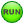(Run) button.
5. In the panel, change the End time to 5.0 and the Print interval to 0.01.
6. Export the MotionSolve file, in the menu bar click File > Export > Solver Deck.
Note: Currently there is no GUI option available to specify the DMPSUB file defining flexbody damping, therefore the dmp_nonlin.py must be manually added to the MotionSolve file (*.xml) by adding these statements:
is_user_damp = "TRUE"
usrsub_param_string = "USER()"
interpreter = "Python"
script_name = "dmp_nonlin.py"
usrsub_fnc_name = "DMPSUB"
Your flexbody definition should look like the following example:
<Body_Flexible
id = "30104"
lprf_id = "30104002"
mass = "7.424574879203591E-02"
inertia_xx = "1.471824534365642E+02"
inertia_yy = "4.505004745855096E+00"
inertia_zz = "1.501914135052064E+02"
inertia_xy = "-5.546373592613223E-03"
inertia_yz = "-1.984540442733755E-03"
inertia_xz = "1.557626595859531E-03"
cm_x = "1.191728011928773E-03"
cm_y = "-2.225471002399553E+01"
cm_z = "5.469513396916666E-05"
h3d_file = "conrod.h3d"
is_user_damp = "TRUE"
usrsub_param_string = "USER()"
interpreter = "Python"
script_name = "dmp_nonlin.py"
usrsub_fnc_name = "DMPSUB"
flexdata_id = "30104"
animation_scale = "1."
/>